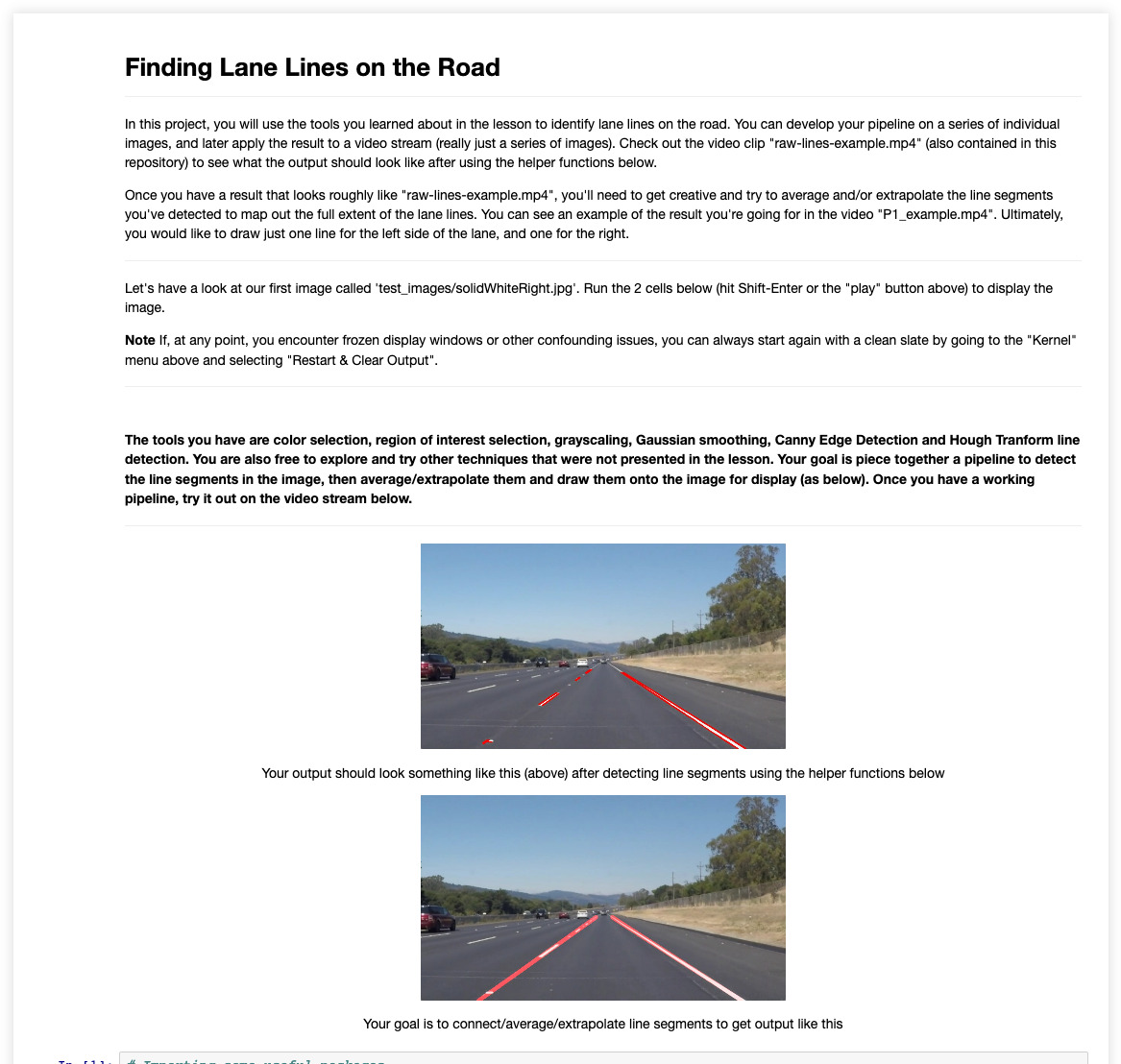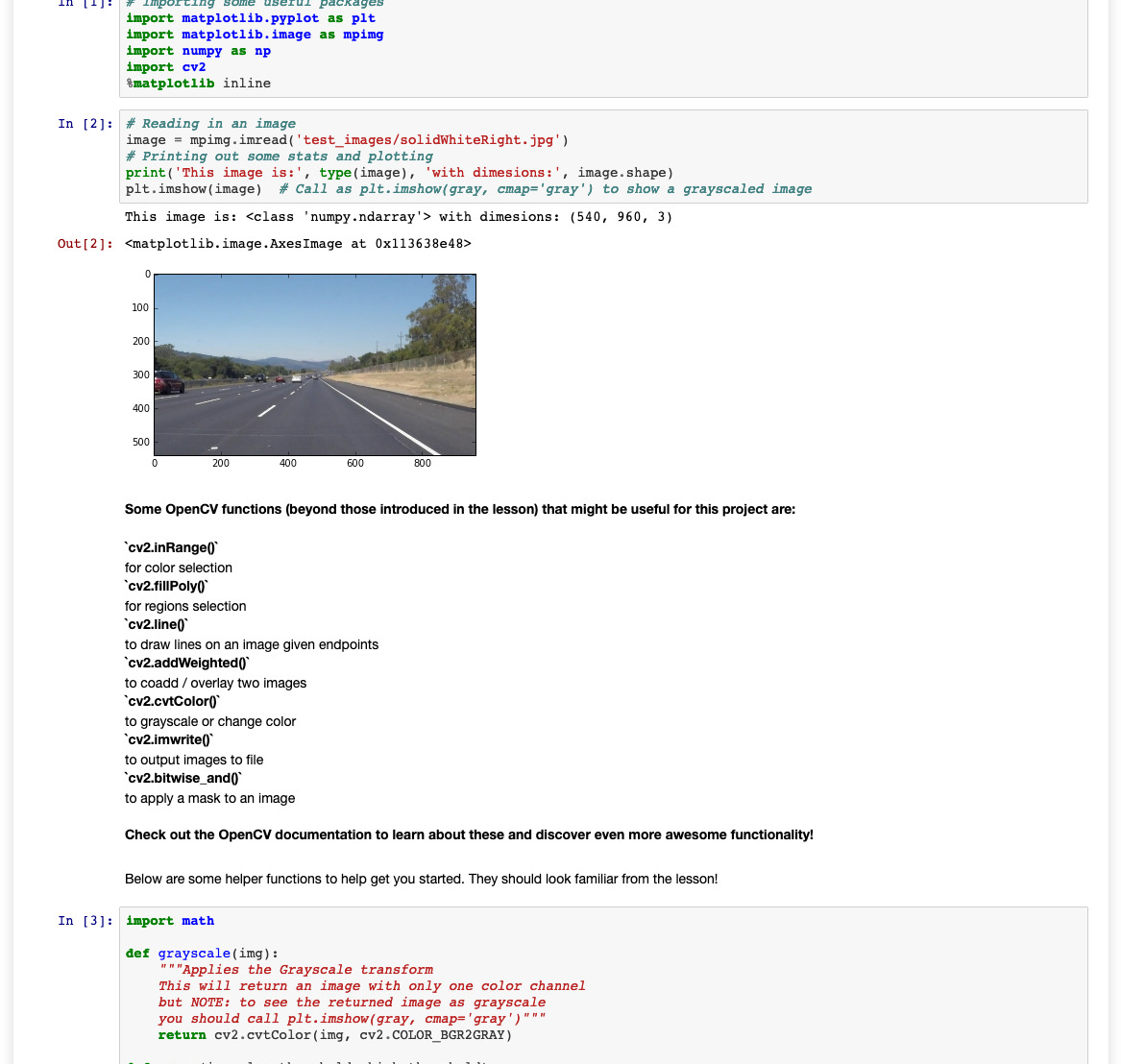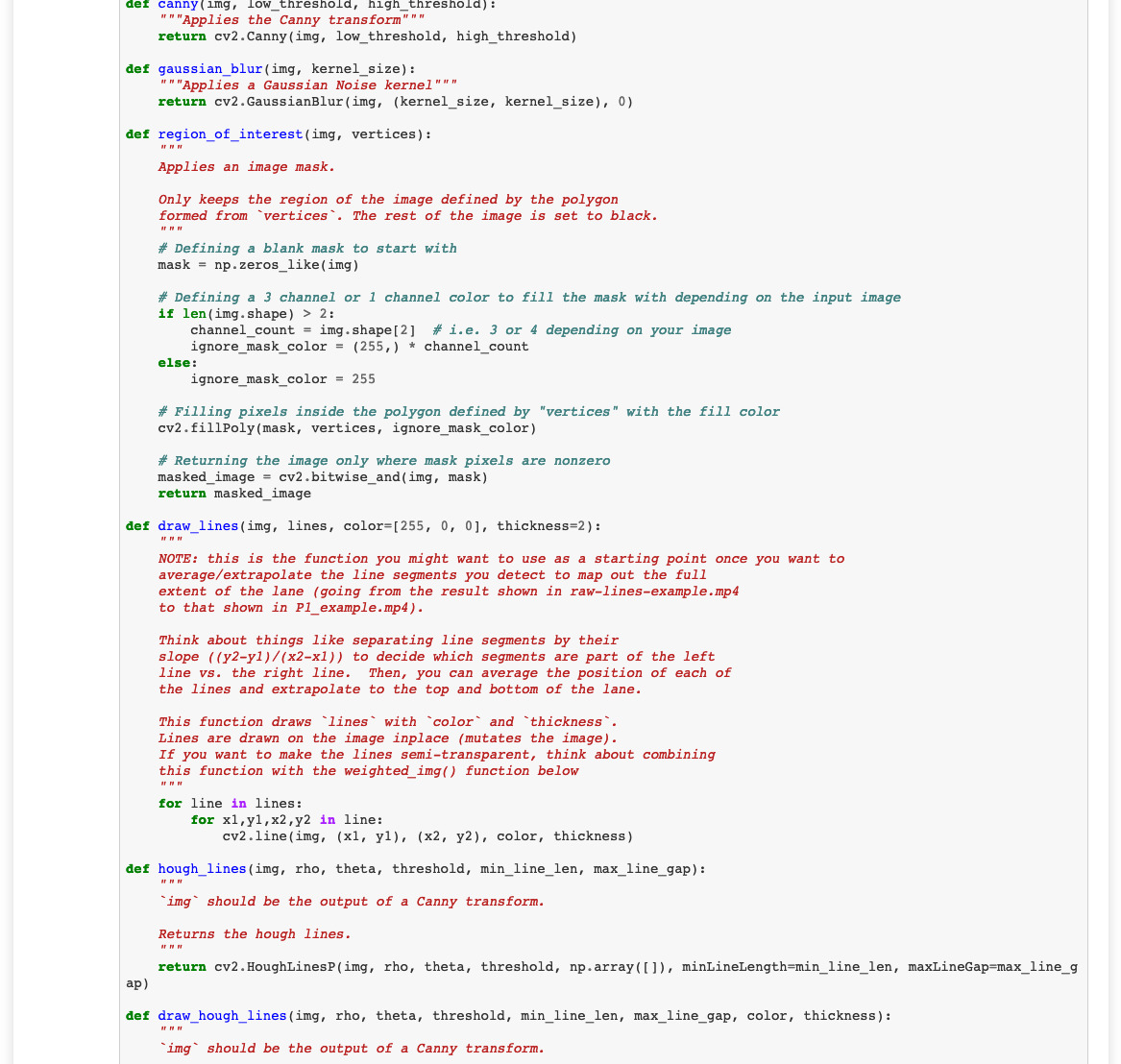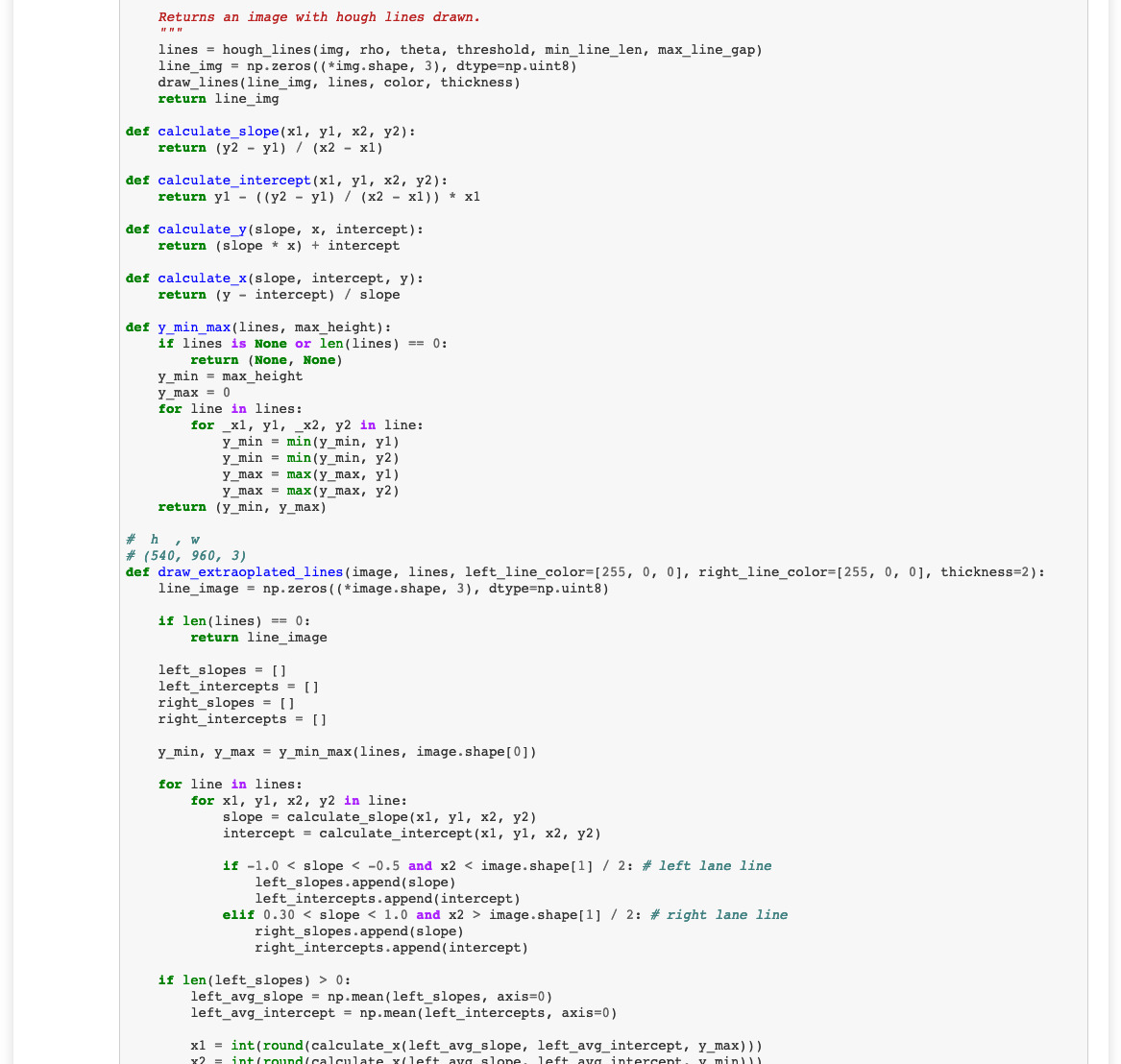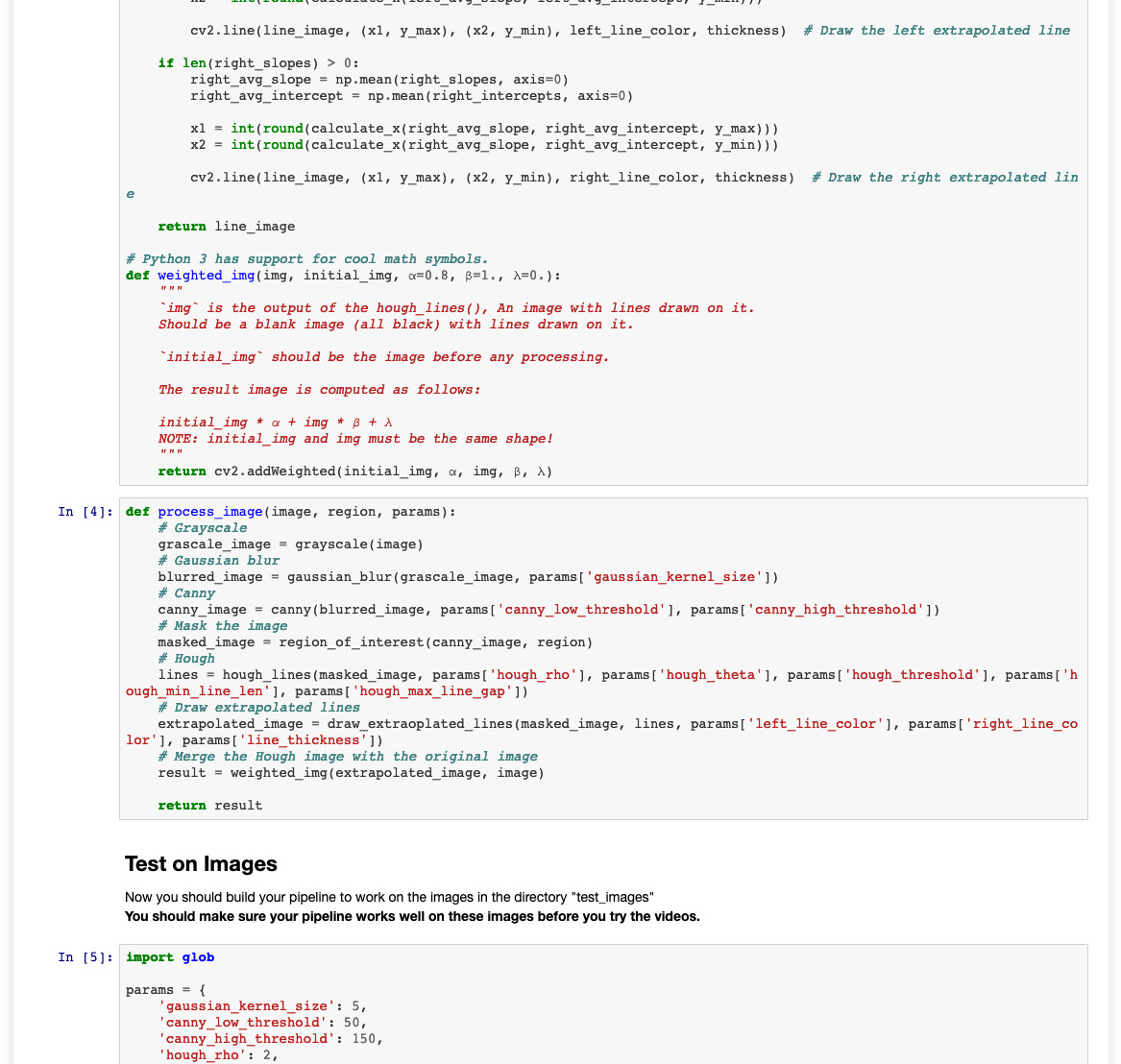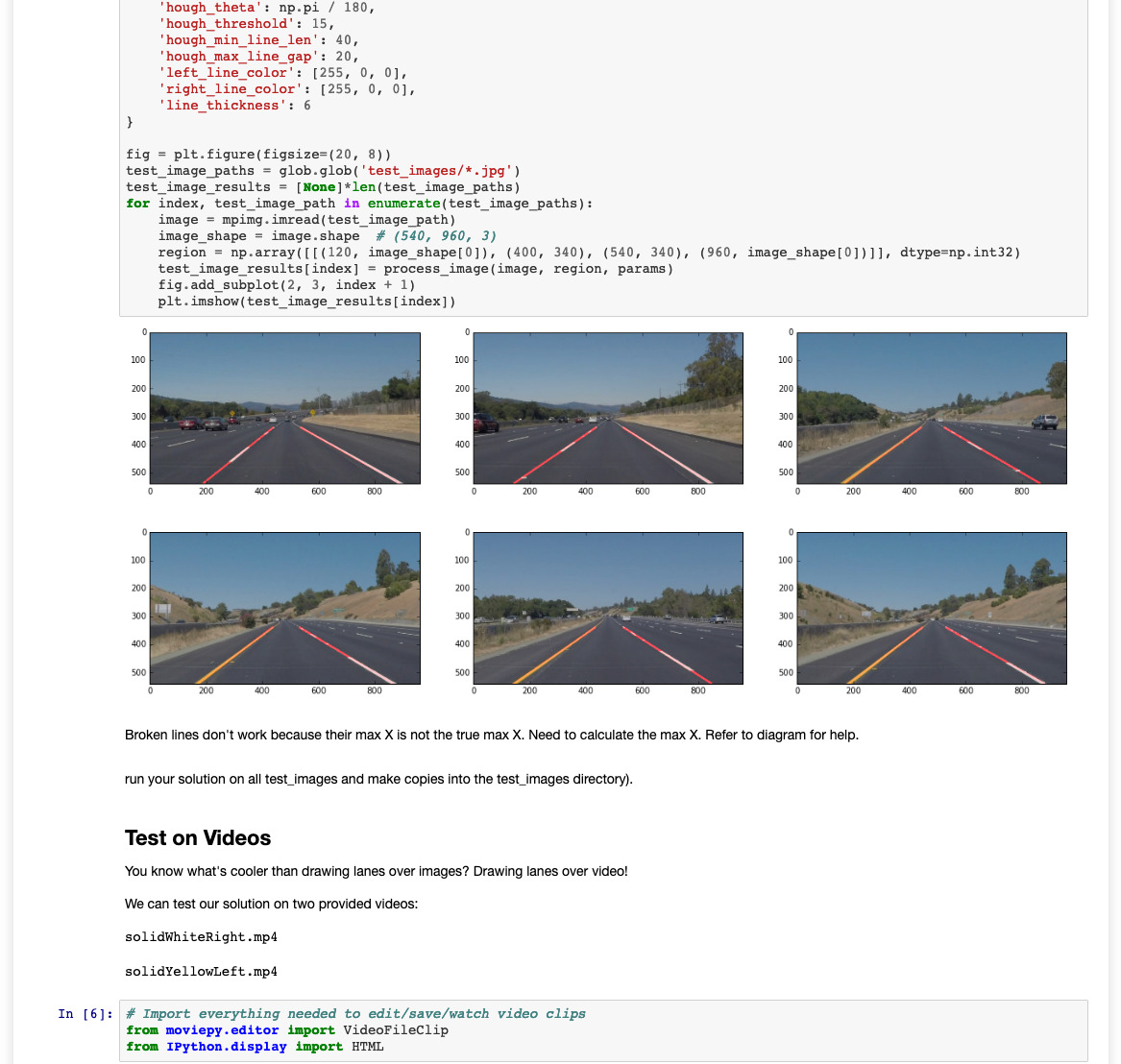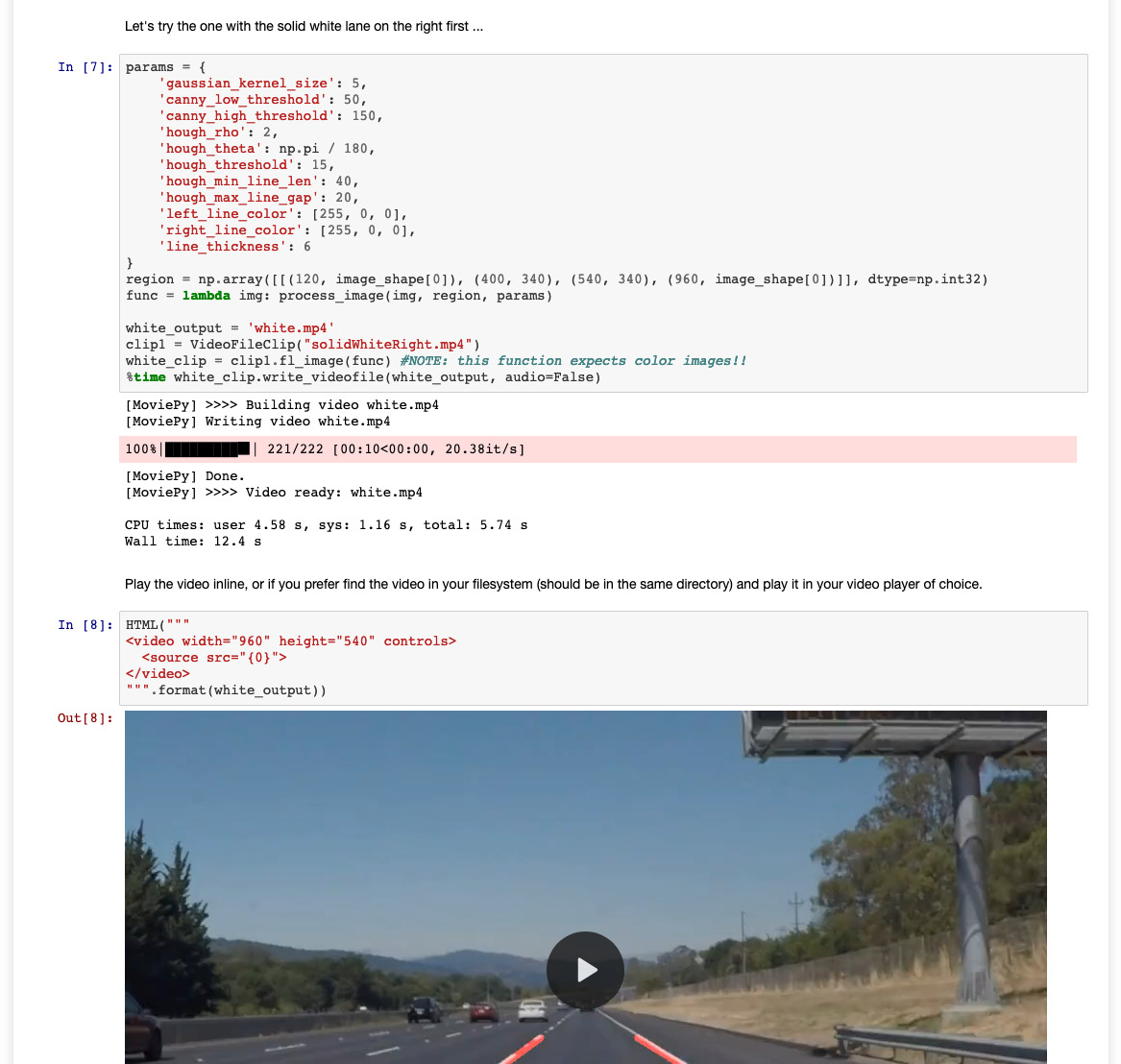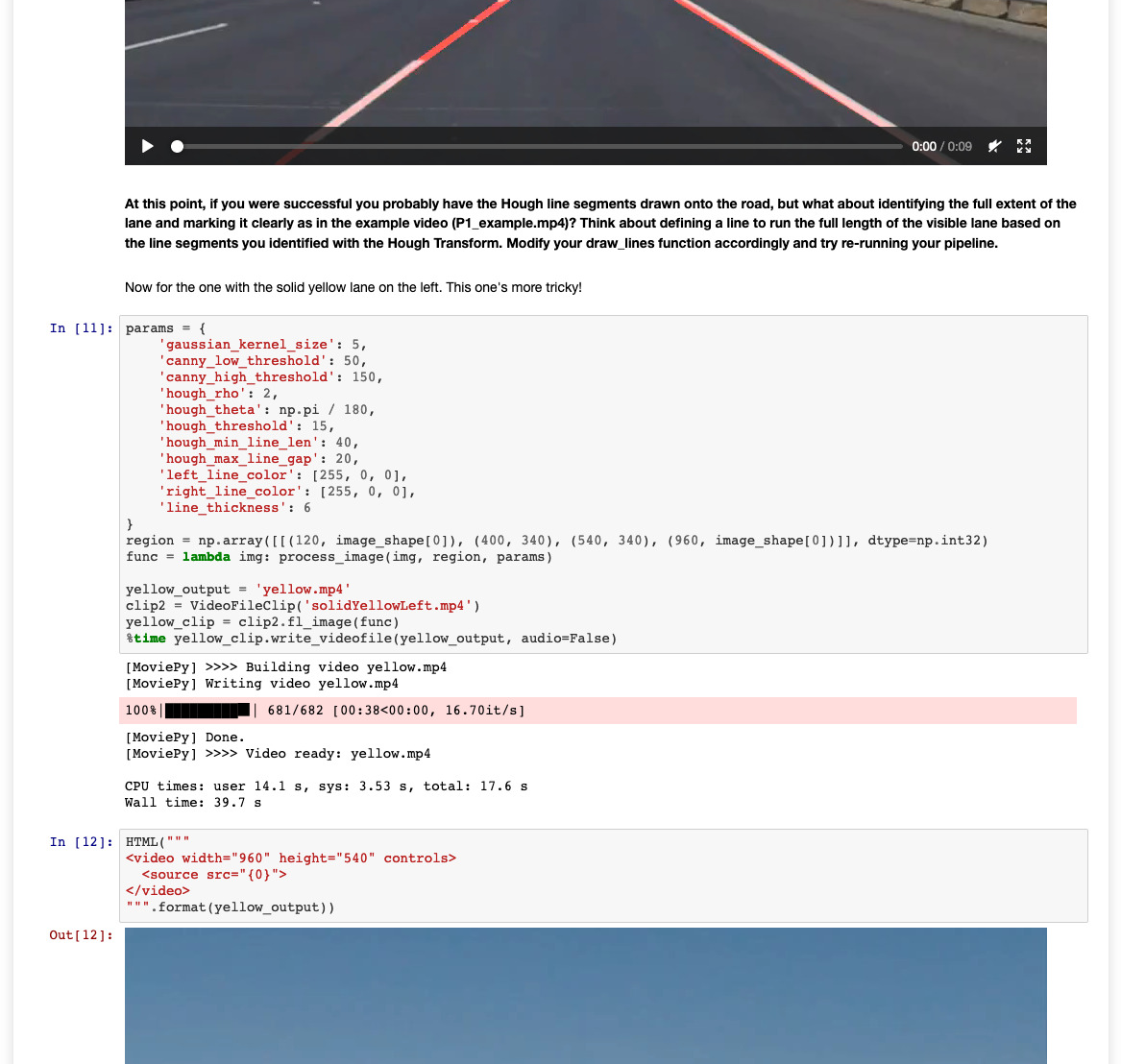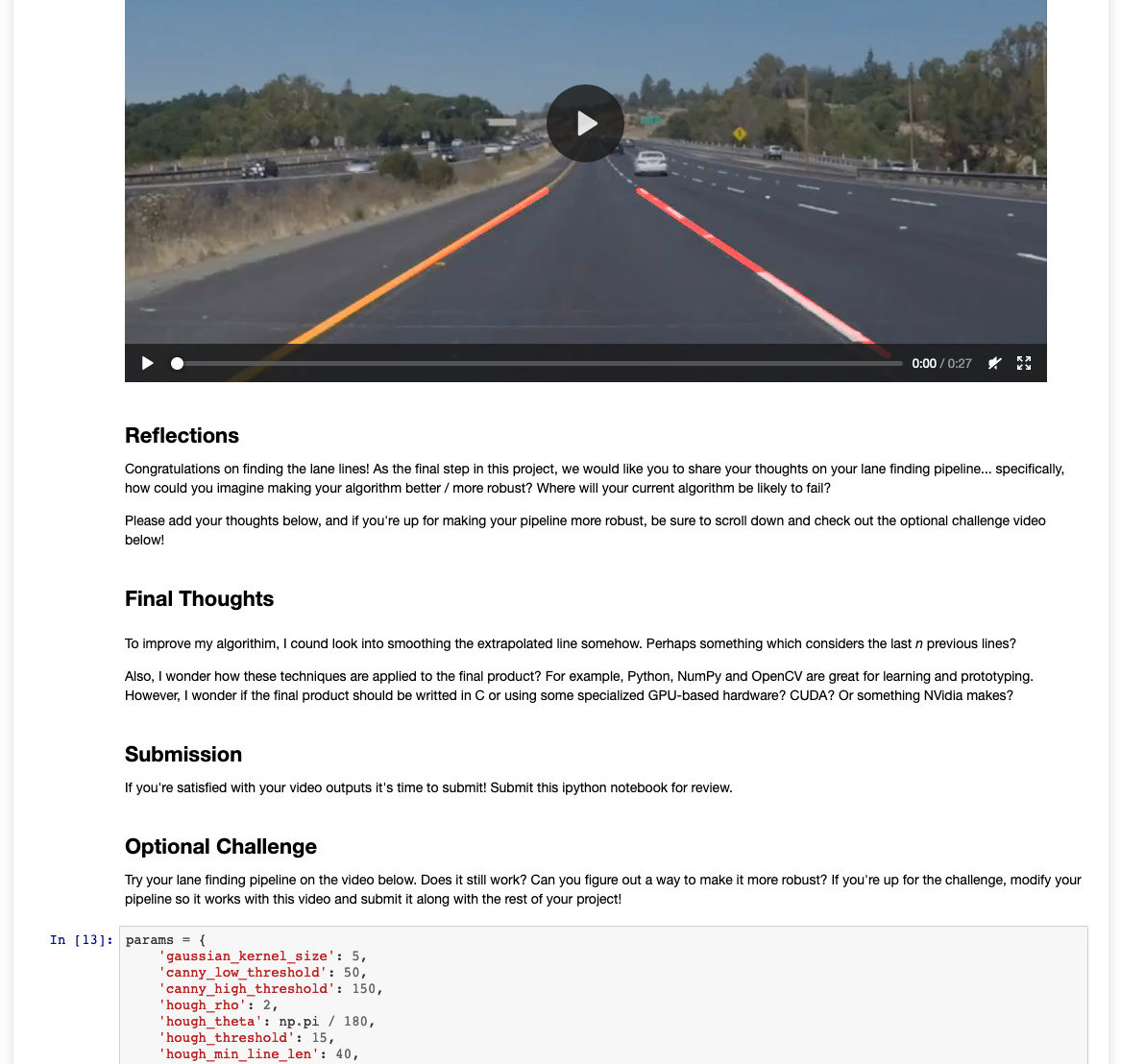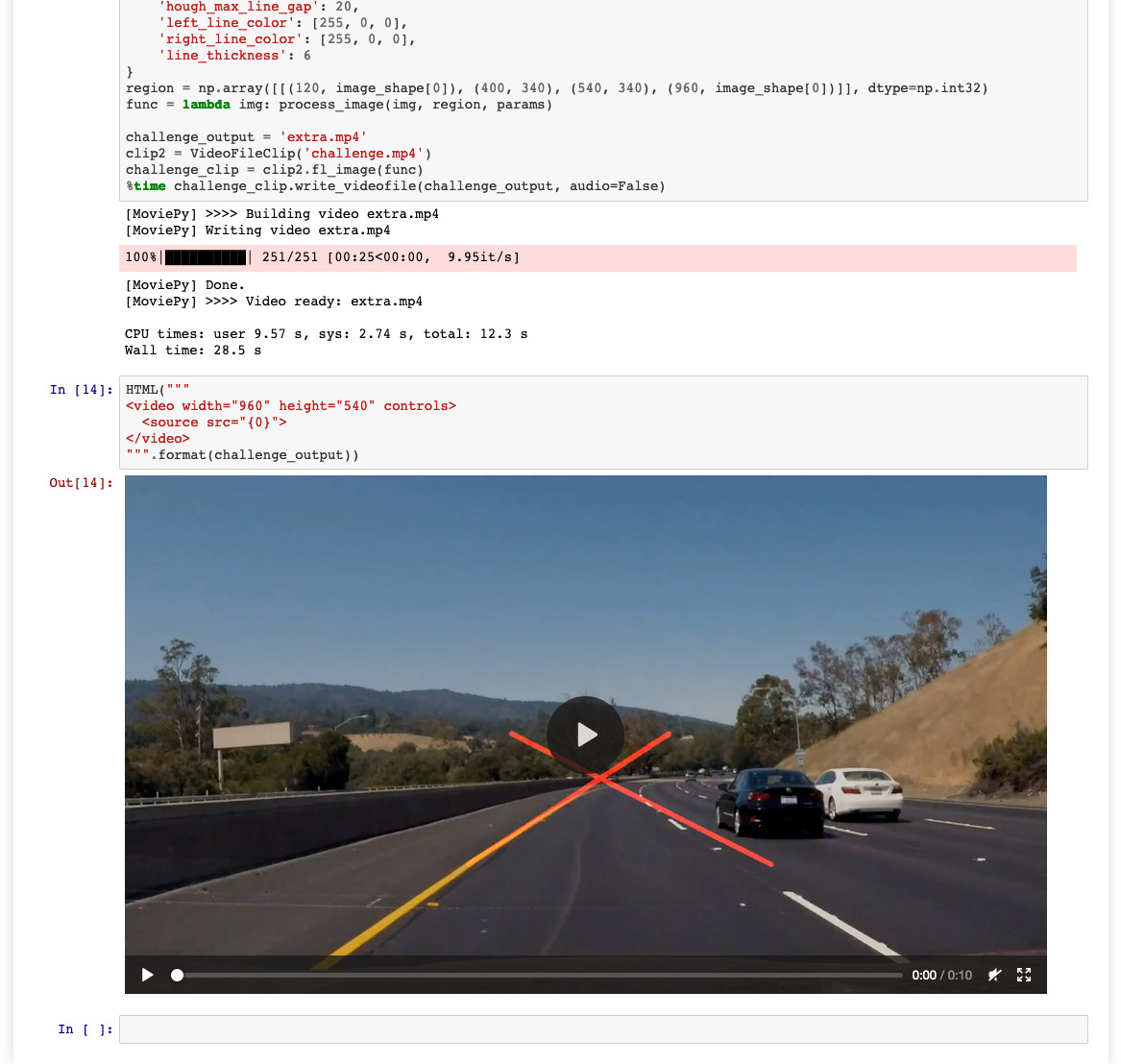# 🛣 Lane Detection using Python

3120 views

Code I wrote in Python utilizing NumPy, OpenCV, etc., for Udacity's Self-Driving Car course. The flow goes like this:

Each video frame is processed as an image:

1. Convert image to grayscale
2. Apply a Gaussian blur to the grayscaled image
3. Generate an image which only contains edges from the grayscaled image (Canny edge detection algorithm)
4. Mask edges image to a region limited to where the camera would be positioned (a triangle in the middle)
5. Determine coordinates of lines from the masked image (Hough transform algorithm)
6. Extrapolate lines:
1. Calculate min ys and max ys from lines
2. Separate lines by slope, into left lines and right lines
3. Calculate mean slope and intercept of left and right lines
4. Calculate extrapolated line for each left and right lines
5. Draw semi-transparent lines onto original image

Repeat for each video frame